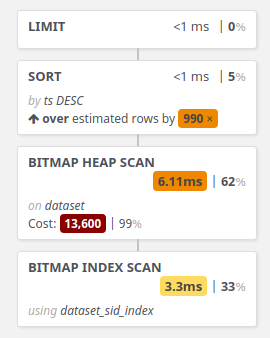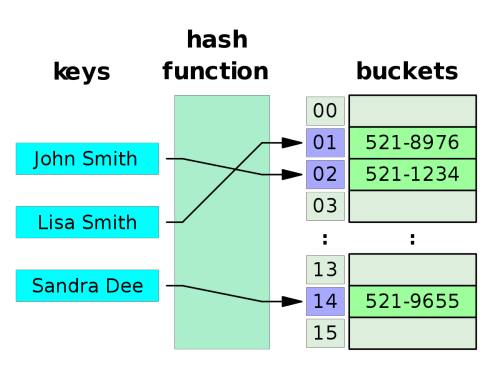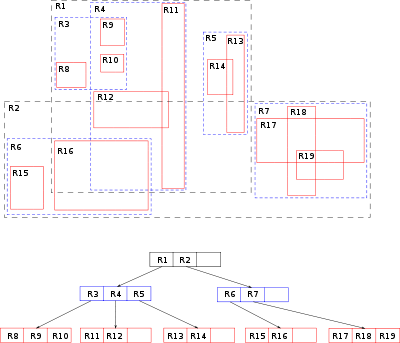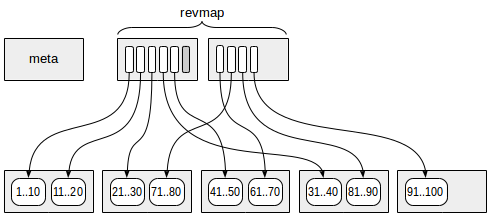# Postgres Indexes

## Deep dive

In this guide we will talk about types of indexes, performance boosting and price of improvements.

Index is addition data structure that allow to support uniqueness or fast access to data. All the time when we create new index we can improve read speed but always we decrease write speed (update index) and increase disk consumption (store index). Index can use multiple columns (or expression) but not all indexes supports this. Someone supports customization parameters.

Memory usage. We can measure memory usage using `pg_relation_size`. All the time when we want to use yet another new index we shall remember about index size. When table is large index can be large too.

``````# SELECT pg_size_pretty(pg_relation_size('index_name'));
pg_size_pretty
----------------
118 MB
(1 row)

# SELECT pg_size_pretty(pg_relation_size('table_name'));
pg_size_pretty
----------------
274 MB
(1 row)``````

Read speed. When new index created we have to verify that target our queries use this index. Remember that sometimes optimizer can ignore index. For this needs use `EXPLAIN (ANALYSE, BUFFERS)` commands.

``````EXPLAIN (ANALYSE, BUFFERS)
SELECT *
FROM dataset
WHERE s_id = 3
ORDER BY ts DESC
LIMIT 5;``````
``````                                                                 QUERY PLAN
---------------------------------------------------------------------------------------------------------------------------------------------
Limit  (cost=13746.95..13746.97 rows=5 width=20) (actual time=9.917..9.917 rows=5 loops=1)
Buffers: shared hit=5333
->  Sort  (cost=13746.95..13759.38 rows=4971 width=20) (actual time=9.915..9.915 rows=5 loops=1)
Sort Key: ts DESC
Sort Method: top-N heapsort  Memory: 25kB
Buffers: shared hit=5333
->  Bitmap Heap Scan on dataset  (cost=94.96..13664.39 rows=4971 width=20) (actual time=3.885..9.413 rows=5745 loops=1)
Recheck Cond: (s_id = 3)
Heap Blocks: exact=5312
Buffers: shared hit=5330
->  Bitmap Index Scan on dataset_sid_index  (cost=0.00..93.72 rows=4971 width=0) (actual time=3.300..3.300 rows=5745 loops=1)
Index Cond: (s_id = 3)
Buffers: shared hit=18
Planning Time: 0.519 ms
Execution Time: 9.998 ms
(15 rows)``````Partial indexes. We found that index size can be large. What we can do with this problem? Use index for part of table. For example we can say that index will be used only for records where ```year >= 2020```. In this case access to `year < 2020` will be slow but it might be ok.

``CREATE INDEX dataset_sid_index_v2 ON dataset (s_id) where ts > 10000;``

Indexes on expressions. We can use expression for indexes. It might be useful when you are using navigation over JSONB field. So you can use any expression without `CURRENT TIME` calls for indexes.

``CREATE INDEX documents_inner_id_index ON documents ( ((data->'inner_id')::int) );``

Cluster table. We can use `CLUSTER` command for table rewriting in specific order. We can expect that table's rows will be use order from index that we used for this command. But before start this command remember that table will be block when operation is running.

``CLUSTER dataset USING dataset_sid_index;``

We can find available indexes in `pg_am` table:

``SELECT * FROM pg_am;``
``````amname |  amhandler  | amtype
--------+-------------+--------
btree  | bthandler   | i
hash   | hashhandler | i
gist   | gisthandler | i
gin    | ginhandler  | i
spgist | spghandler  | i
brin   | brinhandler | i
(6 rows)``````

# Indexes

## BTree## Hash index## GiST index## GIN index## SP-GiST index## BRIN index## RUM index

Related articles:Author @mrkandreev

Machine Learning Engineer